### Dual Nature Of Radiation And Matter Class 12th Physics Part Ii CBSE Solution

##### Question 1.(a) Estimate the speed with which electrons emitted from a heated emitter of an evacuated tube impinge on the collector maintained at a potential difference of 500 V with respect to the emitter.Ignore the small initial speeds of the electrons. The specific charge of the electron, i.e., its e/m is given to be 1.76 × 1011 C kg–1.(b) Use the same formula you employ in (a) to obtain electron speed for a collector potential of 10 MV. Do you see what is wrong? In what way is the formula to be modified?Answer:Given:Potential difference between collector and emitter = 500VSpecific charge of electron (charge per unit mass e/m) = 1.76 × 1011 CKinetic energy of an electron is given by: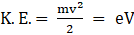…(1)Where,M = mass of electronv = velocity of electrone = charge of electronV = potential difference (accelerating potential)(a) From equation (1), we can write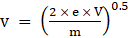…(2)By putting the values in equation (2) we can find electron velocity.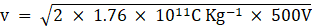v = 1.327 × 107 ms-1(b) Accelerating potential, V = 10MV = 106VLet speed of electron be v1Again putting the values in equation (2),v1 =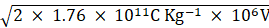v1 = 1.8 × 109ms-1This result is wrong as we understand that speed of light(i.e. 3 × 108 ms-1) is the theoretical limit of the speed.Such problems can be dealt using relativistic mechanics,Relativistic mass is given by:m =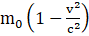Where,m = relativistic massm0 = rest massv = velocity of particlec = speed of lightAt relativistic speeds, kinetic energy is given by,KE = mc2-m0c2Question 2.(a) A monoenergetic electron beam with electron speed of 5.20 × 106 m s–1 is subject to a magnetic field of 1.30 × 10–4 T normal to the beam velocity. What is the radius of the circle traced by the beam, given e/m for electron equals 1.76 × 1011C kg–1.(b) Is the formula you employ in (a) valid for calculating the radius of the path of a 20 MeV electron beam? If not, in what way is it modified?[Note: Exercises 11.20(b) and 11.21(b) take you to relativistic mechanics which is beyond the scope of this book. They have been inserted here simply to emphasize the point that the formulas you use in part (a) of the exercises are not valid at very high speeds or energies. See answers at the end to know what ‘very high speed or energy’ means.]Answer:Given:Speed of electron, v = 5.20 × 106 ms-1Magnetic field strength normal to the beam = 1.30 × 10–4 TCharge to mass ratio (e/m) = 1.76 × 1011 C Kg-1(a) Force applied by magnetic field,F = e|v × B|F = e × v × B × sinθ …(1)Where,e = Charge on electronv = velocity of particleB = magnetic field strengthθ = angle between Magnetic field and velocitySince the electron traces a circular path, we can use the following equation of centrifugal force:F =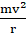…(2)Where,m = mass of particlev = velocity of particler = radius of circle tracedBy equating (1) and (2), we get,evB × sin90° =r =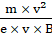→ r =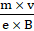…(3)→ r =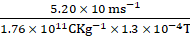→ r = 22.7 6 cm(b) Energy of the electron beam, E = 20 MeVE = 20 × 106 × 1.6 × 10-19JThe energy of an electron is given by:E =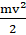From the above equation, we can write,v =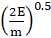…(4)putting the value in equation (4)v =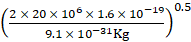v = 2.6 × 109 ms-1This result is incorrect because the speed of any massive object can’t exceed the speed of light (i.e. 3 × 108 ms-1). We can’t use equation (4) in the case where the speed is relativistic:At relativistic speeds, mass is given by,m =Where,m = relativistic massm0 = rest massv = velocity of particlec = speed of lightthe radius of the path traced is given by,r =…(3)By substituting the value of relativistic mass in equation (3) we get,r =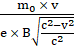…(4)By using equation (4) we can find the radius traced by electrons moving at relativistic speed.By substituting value of relativistic mass in equationQuestion 3.An electron gun with its collector at a potential of 100 V fires out electrons in a spherical bulb containing hydrogen gas at low pressure (∼10–2 mm of Hg). A magnetic field of 2.83 × 10–4 T curves the path of the electrons in a circular orbit of radius 12.0 cm. (The path can be viewed because the gas ions in the path focus the beam by attracting electrons, and emitting light by electron capture; this method is known as the ‘fine beam tube’ method.) Determine e/m from the data.Answer:Given:Potential at collector, V = 100VMagnetic field strength, B = 2.83 × 10-4 TRadius of orbit traced by electron, r = 12.0 cmLet specific charge (i.e. e/m) = KKinetic energy of an electron is given by:KE == eV→ v =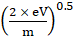…(1)Where,M = mass of electronv = velocity of electrone = charge of electronV = potential difference (accelerating potential)We also know that the bending is caused by the magnetic field,So we can write,Magnetic force = centrifugal forcee × v × B =→ e × B =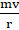→ v =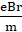…(2)Where,e = Charge on electronv = velocity of particleB = magnetic field strengthm = mass of electronr = radius of trajectoryBy equating (1) and (2) we can write,.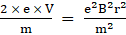→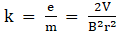By putting the values in above equation we get,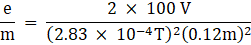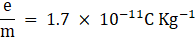Hence the specific charge is 1.7 × 10-11 C Kg-1.Question 4.(a) An X-ray tube produces a continuous spectrum of radiation with its short wavelength end at 0.45 Å. What is the maximum energy of a photon in the radiation?(b) From your answer to (a), guess what order of accelerating voltage (for electrons) is required in such a tube?Answer:Given:The wavelength of radiation produced by tube, λ = 0.45 Åi.e. 0.45 × 10-10 m(a) The maximum energy of the photon is given by,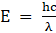…(1)Where,E = Energy of photonh = Planck’s constant = 6.6 × 10-34 Jsc = 3 × 108 ms-1λ = wavelength of photonby putting the values in equation (1), we get,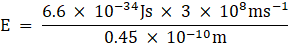E = 44 × 10-16 J or 27.6 × 103 eVThe maximum energy of the photon in x-ray is 44 × 10-16J.(b) For a photon to have 27 KeV of energy, the accelerating potential must be of the order of 30 KeV.Question 5.In an accelerator experiment on high-energy collisions of electrons with positrons, a certain event is interpreted as the annihilation of an electron-positron pair of total energy 10.2 BeV into two γ-rays of equal energy. What is the wavelength associated with each γ-ray? (1BeV = 109 eV)Answer:Given:Total energy of system, E = 10.2 BeV = 10.2 × 109 × 1.6 × 10-19JE = 16.32 × 10-10JEnergy in each ray, E’ = E/2E’ = 8.16 × 10-10JWe know that Energy in a photon is given by,…(1)Where,E = Energy of photonh = Planck’s constant = 6.6 × 10-34 Jsc = 3 × 108 ms-1λ = wavelength of photonFrom equation (1) we can get,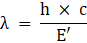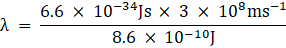λ = 2.34 × 10-16mHence, the wavelength of each ray is 2.3 × 10-16 m.Question 6.Estimating the following two numbers should be interesting. The first number will tell you why radio engineers do not need to worry much about photons! The second number tells you why our eye can never ‘count photons’, even in the barely detectable light.(a) The number of photons emitted per second by a Medium wave transmitter of 10 kW power, emitting radio waves of wavelength 500 m.(b) The number of photons entering the pupil of our eye per second corresponding to the minimum intensity of white light that we humans can perceive (∼10–10 W m–2). Take the area of the pupil to be about 0.4 cm2, and the average frequency of white light to be about 6 × 1014 Hz.Answer:Given:Power of transmitter, E’ = 10 KW = 10000 Js-1Wavelength of radio waves emitted = 500 m(a) We know that Energy in a wave is given by,…(1)Where,E = Energy of photonh = Planck’s constant = 6.6 × 10-34 Jsc = 3 × 108 ms-1λ = wavelength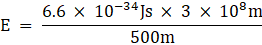E = 3.9 × 10-28 JTotal power = number of photons emitted × Energy of photonE’ = n × En =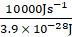n = 2.55 × 1031 s-1n = 3 × 10-31 s-1Here we see that the number of radio waves emitted per second is very high.(b) Given:Intensity of light perceived by human eyes, I = 10-10Wm-2Area of pupil, A = 0.4 × 10-4 m2Frequency of white light, v = 6 × 1014HzThe energy of each photon is given by,E = hvWhere,E = energy of photonh = Planck’s constant = 6.6 × 10-34Jsv = 6 × 1014HzE = 6.6 × 10-34Js × 6 × 1014 s-1E = 3.96 × 10-19JEnergy of each photon is 3.96 × 10-19JLet total number of photons being emitted per second, falling on unit area = nWe define intensity as the amount of energy falling on unit area in unit time so we can write,I = n × 3.96 × 10-19J10-10 Jm-2s-1 = n × 3.96 × 10-19Jn = 2.52 × 108 m-2s-1Number of photons entering pupil = area of pupil × nNumber of photons entering pupils = 0.4 × 10-4m2 × 2.52 × 108 m-2s-1→ np = 1.0082 × 104s-1Almost 10000 photons enter our pupil per second.Question 7.Ultraviolet light of wavelength 2271 Å from a 100 W mercury source irradiates a photo-cell made of molybdenum metal. If the stopping potential is –1.3 V, estimate the work function of the metal. How would the photo-cell respond to a high intensity (∼105 W m–2) red light of wavelength 6328 Å produced by a He-Ne laser?Answer:Given:Wavelength of light, λ = 2271 Å = 2271 × 10-10mPower of mercury source, E = 100 Js-1Stopping potential, Vs = -1.3 VLet frequency of light = vWork function, Φ0 is given by,Φ0 = hv-eVsΦ0 =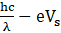Where,h = Planck’s constant = 6.6 × 10-34Jsc = speed of light = 3 × 108mλ = wavelength of lighte = charge on each electron = 1.6 × 10-19CΦ0 =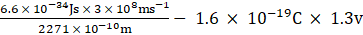Φ0 = 6.64 × 10-19JΦ0 = (6.64/1.6) × 10-19 eV = 4.15 eVLet v0 be the threshold frequency of the metal,Φ0 = hv0v0 = Φ0/h→ v0 = 6.6 × 10-19/ 6.6 × 10-34→ v0 = 1.00. × 10-15 s-1Wavelength of red light, λ’ = 6323 × 10-10mFrequency of red light can be given as,v' = c/λ’v’ =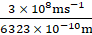v’ = 4.74 × 1014 HzSince the threshold frequency is greater than the frequency of red light, the photocell will not respond to the red light produced.Question 8.Monochromatic radiation of wavelength 640.2 nm (1nm = 10–9 m) from a neon lamp irradiates photosensitive material made of caesium on tungsten. The stopping voltage is measured to be 0.54 V. The source is replaced by an iron source and its 427.2 nm line irradiates the same photo-cell. Predict the new stopping voltage.Answer:Given:Wavelength of light, λ = 640.2 nm = 640.2 × 10-9mStopping potential, V0 = 0.54 VLet frequency of light = vWork function, Φ0 is given by,Φ0 = hv-eVsΦ0 =…(1)Where,h = Planck’s constant = 6.6 × 10-34Jsc = speed of light = 3 × 108mλ = wavelength of lighte = charge on each electron = 1.6 × 10-19CΦ0 =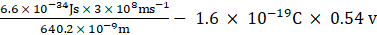Φ0 = 2.229 × 10-19J or 1.39 eVWavelength of radiation emitted by iron, λ’ = 427.2 nmλ’ = 4.27.2 × 10-9mThe new stopping potential can be found using equation (1),Let new stopping potential = v’By putting the values in equation (1) we get,Φ0 =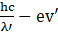→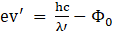→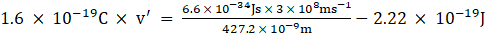→ v’ = 1.5 eVTherefore, the new stopping potential is 1.5 eV.Question 9.A mercury lamp is a convenient source for studying frequency dependence of photoelectric emission since it gives a number of spectral lines ranging from the UV to the red end of the visible spectrum. In our experiment with rubidium photo-cell, the following lines from a mercury source were used:λ1 = 3650 Å, λ2 = 4047 Å, λ3 = 4358 Å, λ4 = 5461 Å, λ5 = 6907 Å,The stopping voltages, respectively, were measured to be:V01 = 1.28 V, V02 = 0.95 V, V03 = 0.74 V, V04 = 0.16 V, V05 = 0 VDetermine the value of Planck’s constant h, the threshold frequency and work function for the material.[Note: You will notice that to get h from the data, you will need to know e (which you can take to be 1.6 × 10–19 C). Experiments of this kind on Na, Li, K, etc. were performed by Millikan, who, using his own value of e (from the oil-drop experiment) confirmed Einstein’s photoelectric equation and at the same time gave an independent estimate of the value of h.]Answer:Given:The frequency, v of a wave with wavelength λ is given by,v = c/λ …(1)(c = speed of light = 3 × 108ms-1)By using equation (1) we can find frequency for each case,The equation for photo-electric effect is given as,Φ0 = hv- eV0We can rewrite this equation as,V0 =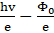…(2)Where,h = Planck’s constantc = speed of light = 3 × 108mλ = wavelength of lighte = charge on each electron = 1.6 × 10-19C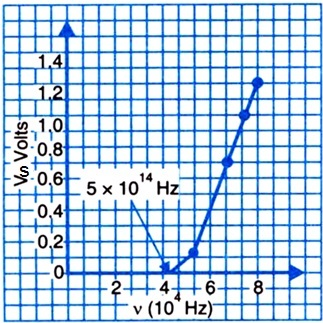We find that the slope of the graph remains same,Slope of the line =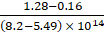From equation (2),We can write,Slope = h/eh = e × slopeh =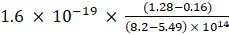h = 6.58 × 10-34 JsFrom the same graph, we can infer that, threshold frequency of the metal is 5 × 1014 Hzi.e. v0 = 5 × 1014HzWork function, Φ0 = hv0 …(2)Where,h = Plank’s constant = 6.57 × 10-34Jsv0 = threshold frequencyBy plugging the data in equation (2), we get,Φ0 = 6.57 × 10-34Js × 5 × 1014Hz→ Φ0 = 3.28 × 10-19 J→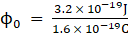→ Φ0 = 2eVHence, the work function of the metal is 2eV.Question 10.The work function for the following metals is given:Na: 2.75 eV; K: 2.30 eV; Mo: 4.17 eV; Ni: 5.15 eV. Which of these metals will not give photoelectric emission for a radiation of wavelength 3300 Å from a He-Cd laser placed 1 m away from the photocell? What happens if the laser is brought nearer and placed 50 cm away?Answer:Given:Wavelength of radiation from laser = 3300 Å = 3300 × 10-10mEnergy radiated by the laser, E is given by,…(1)Where,E = Energy of photonh = Planck’s constant = 6.6 × 10-34 Jsc = 3 × 108 ms-1λ = wavelength of photonputting values in equation (1) we get,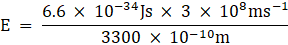E = 6 × 10-19 JE = (6/1.6) × 10-19E = 3.158 eVWe observe that Na and K have work functions less than the energy radiated by the laser so photoelectric effect will take place in case of them, whereas no photo-electric effect will occur in case of Mo and Ni.Even if the laser is brought closer, it will have no effect on the outcome as the photo-electric effect is independent of the intensity of light.Question 11.Light of intensity 10–5 W m–2 falls on a sodium photo-cell of surface area 2 cm2. Assuming that the top 5 layers of sodium absorb the incident energy, estimate time required for photoelectric emission in the wave-picture of radiation. The work function for the metal is given to be about 2 eV. What is the implication of your answer?Answer:Given:Intensity of light, I = 10-5 Wm-2Surface area of photo-cell, A = 2 cm2 = 0.0002 m2Work function of sodium, Φ0 = 2eV = 2 × 1.6 × 10-19 = 3.2 × 10-19JBy knowing the effective area of each sodium atom we can find the absorption of incident energy,Effective area of each sodium atom, ANa = 10-20 m2Number of layers of sodium, n = 5Number of atoms absorbing radiation, NNa = n × (ANa/ A)→ NNa = 5 × (10-20 m2/ 0.0002 m2)→ NNa = 1017So we conclude that 1017 atoms are effectively absorbing radiation.Energy absorbed per atom, E = I/NNaE = 10-5Js-1/1017→ E = 2 × 10-26 Js-1Times required for photo electric emission, t is give by,t = Φ0/Et = 3.2 × 10-19 J / 2 × 10-26 Js-1t = 1.6 × 107 st = 0.5 yearsHence the time requires to initiate photoelectric emission is 0.5 years which is impractical hence, the wave model stands in disagreement with the experimental results.Question 12.Crystal diffraction experiments can be performed using X-rays, or electrons accelerated through appropriate voltage. Which probe has greater energy? (For quantitative comparison, take the wavelength of the probe equal to 1 Å, which is of the order of inter-atomic spacing in the lattice) (me = 9.11 × 10–31 kg).Answer:Given:Wavelength of probe, λ = 1 Å = 10-10mMass of electron, me = 9.11 × 10-31KgThe kinetic energy of an electron is given by: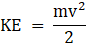We can write,mv = (2 × m × KE)0.5P = (2 × m × KE)0.5 ...(1)Where,m = mass of electronv = velocity of electronp = momentum of particleDe-broglie wavelength is given by,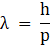Where,λ = Wavelengthh = Planck’s constantp = momentumFrom equation (1) we can write,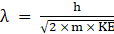…(2)From equation (2) we can write that,KE =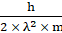Putting the values in above equation we get,KE =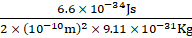KE = 2.39 × 10-17 JKE for an electron is 2.39 × 10-17 J.Now for photon,E’ =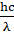…(4)Where,E = energy of photonh = Planck’s constantc = speed of lightλ = wavelength of the photonBy putting values in equation (4 ) we get,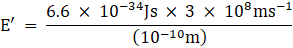E’ = 19.6 × 10-16 JThe photon will have more energy as compared to the accelerated electron for the same wavelength.Question 13.Obtain the de Broglie wavelength of a neutron of kinetic energy 150 eV. As you have seen in Exercise 11.31, an electron beam of this energy is suitable for crystal diffraction experiments. Would a neutron beam of the same energy be equally suitable? Explain.(mn = 1.675 × 10–27 kg)Answer:Given:Kinetic energy of neutron, E = 150 eV = 150 × 1.6 × 10-19E = 2.4 × 10-17 JMass of neutron, mn = 1.675 × 10-27 KgKinetic energy of a particle is given by,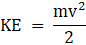We can write,mv = (2 × m × KE)0.5P = (2 × m × KE)0.5 ...(1)Where,m = mass of electronv = velocity of electronp = momentum of particleBy putting the equation (1) in de- Broglie equation, we get,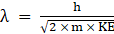…(2)Now substituting the values in eq (2) we get,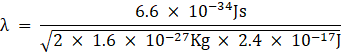λ = 2.327 × 10-12mA neutron cannot be used in diffraction experiment as lattice spaces are of the order of few 10-10 m, whereas the wavelength of a neutron beam is 100 times smaller.Question 14.Obtain the de Broglie wavelength associated with thermal neutrons at room temperature (27°C). Hence explain why a fast neutron beam needs to be thermalised with the environment before it can be used for neutron diffraction experiments.Answer:De- Broglie wavelength, λ = 1.447 × 10-10 mRoom temperature, T = 300KThe average KE of neutron is given as,KE = 3/2 KT …(3)Where,K = Boltzmann constant = 1.38 × 10-23 Jmol-1K-1From equation 2,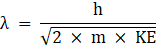Using equation (3) we can write,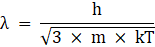By putting values in above equation we get,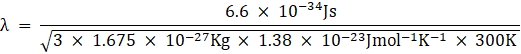λ = 1.447 × 10-10 mNow, the wavelength has come into comparable range with the inter-atomic spaces, it is now suitable for diffraction. Hence a beam should be thermalized before using for diffraction.Question 15.An electron microscope uses electrons accelerated by a voltage of 50 kV. Determine the de Broglie wavelength associated with the electrons. If other factors (such as numerical aperture, etc.) are taken to be roughly the same, how does the resolving power of an electron microscope compare with that of an optical microscope which uses yellow light?Answer:Given:Accelerating voltage, V = 50 KVEnergy of each electron is given by,E = eV …(1)Where,e = Charge on electron = 1.6 × 10-19CV = accelerating VoltagePutting the values in equation (1)E = 1.6 × 10-19C × 50 × 1000VE = 8 × 10-15 JDe Broglie wave length is given by,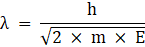Where,m = mass of electronh = Planck’s constant = 6.6 × 10-19 JsE = EnergyBy putting the values in above equation we get,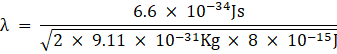λ = 5.4 × 10-12 m.As compared to an optical telescope which uses yellow light, electron microscope has better resolving power (about 105 times better). As the resolving power is inversely proportional to the wavelength of light used.Question 16.The wavelength of a probe is roughly a measure of the size of a structure that it can probe in some detail. The quark structure of protons and neutrons appears at the minute length-scale of 10–15 m or less. This structure was first probed in early 1970’s using high energy electron beams produced by a linear accelerator at Stanford, USA. Guess what might have been the order of energy of these electron beams. (Rest mass energy of electron = 0.511 MeV.)Answer:Given:Order of length of quark structure, λ = 10-15mRest mass energy of electron = 0.511 MeVi.e. m0c2 = 0.511 MeV→ m0c2 = 0.511 × 1.6 × 10-19→ m0c2 = 0.817 × 10-13 JBy using De-Broglie wavelength equation we can write,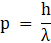Where,p = momentum of particleh = Planck’s constant = 6.6 × 10-34Jsλ = wavelengthThe equation of energy at relativistic speed is,E2 = p2c2 + m20c4E2 = (6.6 × 10-19 × 3 × 108)2 + (0.817 × 10-13)2→ E = (392.07 × 10-22)0.5→ E = 1.9 × 10-10JHence the energy of electron emitted from linear accelerator is 1.9 × 10-10J.Question 17.Find the typical de Broglie wavelength associated with a He atom in helium gas at room temperature (27 °C) and 1 atm pressure; and compare it with the mean separation between two atoms under these conditions.Answer:Given:Pressure, P = 1 atm = 101325 PaTemperature, T = 27° = 300KDe-Broglie wavelength is given by,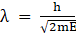…(1)Where,h = Planck’s constant = 6.6 × 10-34 Jsm = mass of HeE = total energyMass of helium, m = Atomic mass/ number of atomsLet there be one mole of He,mass of 1 mol of He = 4 gThen,m = 4/ 6.023 × 1023m = 6.64 × 10-24 = 6.64 × 10-27Kg …(2)We know that average energy at temperature T is given by,E = 3/2 kT …(3)Where,k = Boltzmann constant = 1.38 × 10-23 Jmol-1K-1T = absolute temperatureUsing the equation (3), we can rewrite equation (1) as,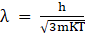…(4)Putting the values in equation (4) we get,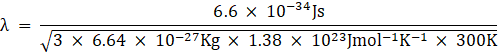λ = 0.72 × 10-10mUsing ideal gas equation we have,PV = RTPV = kNT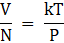Where,V = volumeN = number of molesP = pressurek = Boltzmann constantT = absolute temperatureMean distance between, r is given by,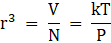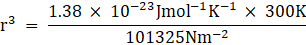r = 3.3 × 10-9mThe mean separation between the atoms is greater than the De- Broglie wavelength.Question 18.Compute the typical de Broglie wavelength of an electron in a metal at 27 °C and compare it with the mean separation between two electrons in a metal which is given to be about 2 × 10–10 m.[Note: Exercises 11.35 and 11.36 reveal that while the wave-packets associated with gaseous molecules under ordinary conditions are non-overlapping, the electron wave-packets in a metal strongly overlap with one another. This suggests that whereas molecules in an ordinary gas can be distinguished apart, electrons in a metal cannot be distinguished apart from one another. This indistinguishability has many fundamental implications which you will explore in more advanced Physics courses.]Answer:Given:Temperature, T = 27° C = 300 KMean separation between electrons, r = 2 × 10-10 mDe-Broglie wavelength of electron is given by,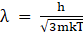…(1)Where,h = Planck’s constant = 6.6 × 10-34 Jsm = mass of electron = 9.1 × 10-31 Kgk = Boltzmann constant = 1.38 × 10-23 Jmol-1K-1T = absolute temperaturePutting the values in equation(1), we get,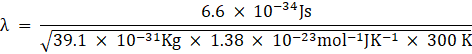λ = 6.2 × 10-9 mThe De-Broglie wavelength is much larger than the interelectronic separation.Question 19.Answer the following question:Quarks inside protons and neutrons are thought to carry fractional charges [( + 2/3)e ; (–1/3)e]. Why do they not show up in Millikan’s oil-drop experiment?Answer:Quarks inside protons and neutrons are known to have fractional charges. Quarks do not show up in Millikan's oil drop experiment as these fractional charges do not exist independently, rather held together by strong nuclear force. These fractional charges are not found free in nature.Question 20.Answer the following question:What is so special about the combination e/m? Why do we not simply talk of e and m separately?Answer:e/m or the specific charge is a important ratio as it is found in basic equations of electro-magnetism, for instance,evB = mv2/r …(1)→ (e/m)vB = v2/rv = Br(e/m)eV = 1/2mv2 …(2)→ v =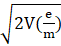We see that the ratio (e/m) has an impact on the dynamics of electron in magnetic field hence e/m is given more importance that e or m.Question 21.Answer the following question:Why should gases be insulators at ordinary pressures and start conducting at very low pressures?Answer:At atmospheric/ ordinary pressures the Ions of gases move randomly and collide with each other , this random motion prevents it from setting a definite current in given direction, at low pressures these random motions decrease and if a potential difference is created between two points the gaseous Ions are able to move in definite direction setting a current. Hence, the gases become conductor at very low pressures.Question 22.Answer the following question:Every metal has a definite work function. Why do all photoelectrons not come out with the same energy if incident radiation is monochromatic? Why is there an energy distribution of photoelectrons?Answer:Work function is the minimum energy required to set off the photo-electric emission. As the incident radiation falls on electrons they absorb defined wavelengths and if they gain enough energy they jump out of metal. But the energy absorption depends is very specific and depends on the initial energy level of electrons. Since all electrons are not in same energy level, all of them can’t absorb the monochromatic wavelength. Since different electrons start from different energy levels so there a energy distribution of photo-electrons.Question 23.Answer the following question:The energy and momentum of an electron are related to the frequency and wavelength of the associated matter wave by the relations: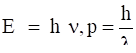But while the value of λ is physically significant, the value of ν (and therefore, the value of the phase speed ν λ) has no physical significance. Why?Answer:We understand that momentum and wavelength of photons have physical significance as it is evident from X-diffraction crystallography. But Energy of photon which is related to the frequency is arbitrary to within an additive constant, hence absolute value of frequency has no physical significance.

PDF FILE TO YOUR EMAIL IMMEDIATELY PURCHASE NOTES & PAPER SOLUTION. @ Rs. 50/- each (GST extra)

HINDI ENTIRE PAPER SOLUTION

MARATHI PAPER SOLUTION

SSC MATHS I PAPER SOLUTION

SSC MATHS II PAPER SOLUTION

SSC SCIENCE I PAPER SOLUTION

SSC SCIENCE II PAPER SOLUTION

SSC ENGLISH PAPER SOLUTION

SSC & HSC ENGLISH WRITING SKILL

HSC ACCOUNTS NOTES

HSC OCM NOTES

HSC ECONOMICS NOTES

HSC SECRETARIAL PRACTICE NOTES

# 2019 Board Paper Solution

HSC ENGLISH SET A 2019 21st February, 2019

HSC ENGLISH SET B 2019 21st February, 2019

HSC ENGLISH SET C 2019 21st February, 2019

HSC ENGLISH SET D 2019 21st February, 2019

SECRETARIAL PRACTICE (S.P) 2019 25th February, 2019

HSC XII PHYSICS 2019 25th February, 2019

CHEMISTRY XII HSC SOLUTION 27th, February, 2019

OCM PAPER SOLUTION 2019 27th, February, 2019

HSC MATHS PAPER SOLUTION COMMERCE, 2nd March, 2019

HSC MATHS PAPER SOLUTION SCIENCE 2nd, March, 2019

SSC ENGLISH STD 10 5TH MARCH, 2019.

HSC XII ACCOUNTS 2019 6th March, 2019

HSC XII BIOLOGY 2019 6TH March, 2019

HSC XII ECONOMICS 9Th March 2019

SSC Maths I March 2019 Solution 10th Standard11th, March, 2019

SSC MATHS II MARCH 2019 SOLUTION 10TH STD.13th March, 2019

SSC SCIENCE I MARCH 2019 SOLUTION 10TH STD. 15th March, 2019.

SSC SCIENCE II MARCH 2019 SOLUTION 10TH STD. 18th March, 2019.

SSC SOCIAL SCIENCE I MARCH 2019 SOLUTION20th March, 2019

SSC SOCIAL SCIENCE II MARCH 2019 SOLUTION, 22nd March, 2019

XII CBSE - BOARD - MARCH - 2019 ENGLISH - QP + SOLUTIONS, 2nd March, 2019

# HSCMaharashtraBoardPapers2020

(Std 12th English Medium)

HSC ECONOMICS MARCH 2020

HSC OCM MARCH 2020

HSC ACCOUNTS MARCH 2020

HSC S.P. MARCH 2020

HSC ENGLISH MARCH 2020

HSC HINDI MARCH 2020

HSC MARATHI MARCH 2020

HSC MATHS MARCH 2020

# SSCMaharashtraBoardPapers2020

(Std 10th English Medium)

English MARCH 2020

HindI MARCH 2020

Hindi (Composite) MARCH 2020

Marathi MARCH 2020

Mathematics (Paper 1) MARCH 2020

Mathematics (Paper 2) MARCH 2020

Sanskrit MARCH 2020

Sanskrit (Composite) MARCH 2020

Science (Paper 1) MARCH 2020

Science (Paper 2)

Geography Model Set 1 2020-2021

MUST REMEMBER THINGS on the day of Exam

Are you prepared? for English Grammar in Board Exam.

Paper Presentation In Board Exam

How to Score Good Marks in SSC Board Exams

Tips To Score More Than 90% Marks In 12th Board Exam

How to write English exams?

How to prepare for board exam when less time is left

How to memorise what you learn for board exam

No. 1 Simple Hack, you can try out, in preparing for Board Exam

How to Study for CBSE Class 10 Board Exams Subject Wise Tips?

JEE Main 2020 Registration Process – Exam Pattern & Important Dates

NEET UG 2020 Registration Process Exam Pattern & Important Dates

How can One Prepare for two Competitive Exams at the same time?

8 Proven Tips to Handle Anxiety before Exams!